# Rates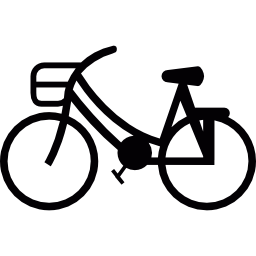You have learned about unit rates. In this lesson, you will improve your understanding of rates by using complex fractions to help you solve different types of problems that involve unit rate comparisons.

Take a look at the problem below using the math you already know to solve the problem.

### Example 1

Marsha is training for a bicycle race that consists of 100 miles. Today, she rode her bike 11 miles in 40 minutes. What is Marsha’s rate in miles per hour?

You know how to use proportions to solve for missing information, so try setting this problem up that way.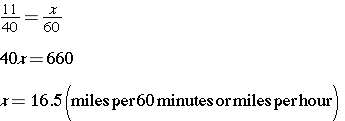The number of miles Marsha bikes in one hour is a unit rate. A unit rate compares two quantities where one of the quantities is 1. It tells you how many units of the first quantity correspond to one unit of the second quantity. The unit rate always has a denominator of 1.

Now, let us solve the problem using complex fractions.

### Example 2

To get the unit rate, simply set up a ratio and divide the numerator by the denominator. In this problem, the units are in miles and hours. This problem states that Marsha bikes 11 miles in 40 minutes. 40 minutes is the same as two thirds of an hour. Since the “per hour” is what you are looking to find, that will be the denominator.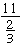is a complex fraction. Remember that a complex fraction is a fraction where the numerator, denominator, or both are fractions. To simplify a complex fraction, you divide just as you would with whole numbers.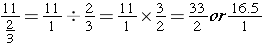The unit rate is 16.5 miles per hour.

### Example 3

On another training ride, Marsha bikes 15 miles in 50 minutes. Using a complex fraction, how could you find the number of miles she bides in 1 hour?

First, you need to represent 50 minutes as a fraction of an hour. 50 minutes is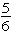of an hour, so that will be your denominator.The unit rate is 18 miles per hour.

Of course, unit rates apply to anything, so let us use complex fractions to find unit rates for price comparisons.

### Example 4

Pat is trying to decide whether or not she should buy a 12-ounce package of coffee on sale for \$7.50 or one-pound of coffee for \$9.00. Which is the better buy?

First, you need to convert 12 ounces to pounds so your rates are the same type of measurement. Then, set up your complex fraction to find out how much a pound of coffee is if you purchase the sale coffee.(12 ounces of coffee is the same as three quarters lb of coffee.)The sale coffee is \$10 per pound and the regular coffee is \$9.00 per pound. The better buy is the one-pound of coffee for \$9.00.

Use what you just learned about unit rates to solve this problem.

Misty’s recipe uses 2 cups of sugar to make two and a half dozen cookies. Rex’s recipe uses two and a quarter cups of sugar to make 3 dozen cookies. Which recipe uses more sugar for a dozen cookies? Hint: Set up complex fractions to solve.

## Unit Rates Practice

1. One of the highest snowfall rates ever recorded was in Silver Lake, Colorado. In April 1921, when just over 7 feet of snow fell in twenty-seven and a half hours. What was that rate in inches per hour?

2. A grocery store sells different types of Trail Mix. Trail Mix A sells for \$6 per three quarters lb, Trail Mix B sells for \$8.50 per lb, and Trail Mix C sells for \$2.25 per 4 oz. Which is the best buy? (There are 16 oz in a lb.)

3. Two friends worked out on treadmills at the gym. Rae walked 2 miles in three quarters of an hour and Grant walked one and three quarters miles in 30 minutes. Who walked at a faster rate?

(source)